# How to use a temperature sensors on a SPI

When using temperature sensors on AVI inputs, the calibration Haltech provides is correct. This is because the AVI's in the Haltech use a 1k pullup to 5 volts.
When using temperature sensors on SPI inputs, the calibration Haltech provides is incorrect. This is because the SPI's in the Haltech use a 10k pull to 5v.
There is a note in the Haltech software about this when you select a SPI for a temperature sensor and click the pull up "Help" question mark.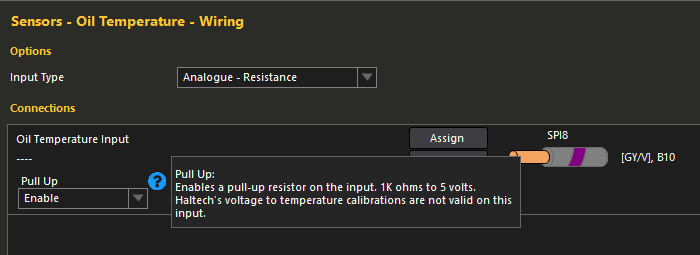We can get around this, with a little math though. We just need to use the Voltage Divider Calculator. You can find them online, I will link the one I like to use below.

First we need to get the calibration of the sensor in resistance, Haltech only provides our sensors in voltage, so we need to convert that first.
For this example, we will use the Haltech oil temperature sensor. I will attach the Haltech calibration info below.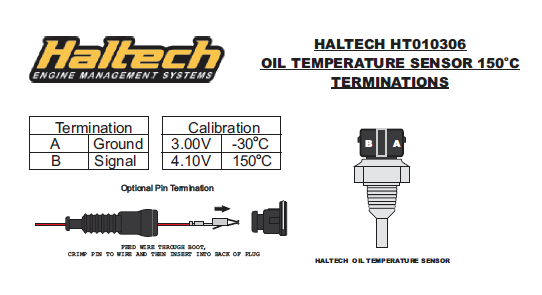So we know that this calibration is in voltage with a 1k pull up to 5 volt, and we need to get it to resistance.
So here is how we will use the calculator.

Voltage source - This is our pull up voltage and in the Haltech it is 5 volts on both AVI's and SPI's.
Resistance 1 - This is the pull up resistance value, so in this case the calibration is given based on a 1k, so we use 1000.
Resistance 2 - This is what we want to figure out so leave it blank.
Output Voltage - This is the number from the Haltech calibration, we will start with 3 volt.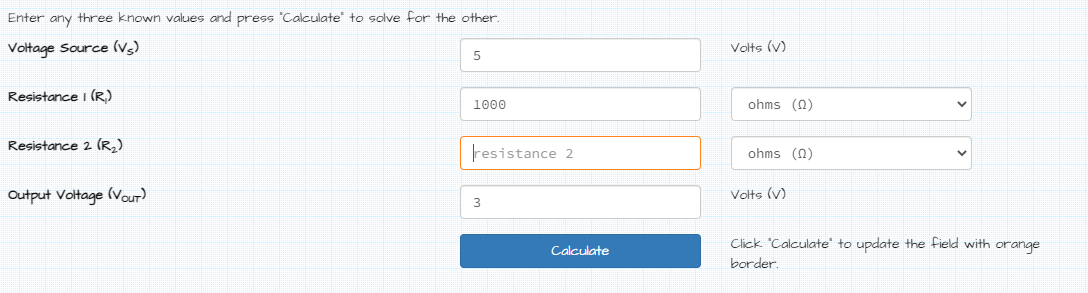Now we click the "Calculate" number and it will fill in the resistance of the sensor at 3v.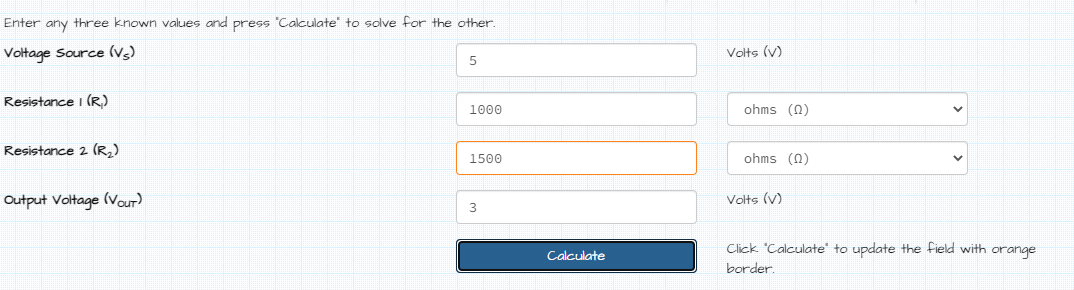Now we know that with a 1k pull up to 5v, the sensor is at 1500ohm when it is -30c.
Do the same for the 4.10 voltage, and you will get 4555.556ohm when the sensor is at 150c.

So the resistance calibration is the following:
1500ohm = -30c
4555.556ohm = 150c

Now that we have the resistance calibration figured out, we can use that to figure out the pull up change from 1k to 10k.
First thing to do is change our calculator to the 10k resistance from the SPI on the resistance 1 box, and clear out the output box. We are going to leave the 1500ohm in the resistance 2 box, because that is the resistance of the sensors at -30c, which is the point we want to find voltage for with the new pull up.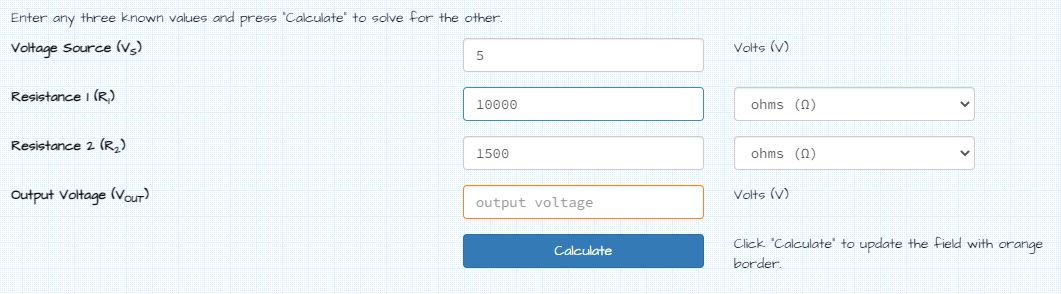Now click calculate, and we will get .652v.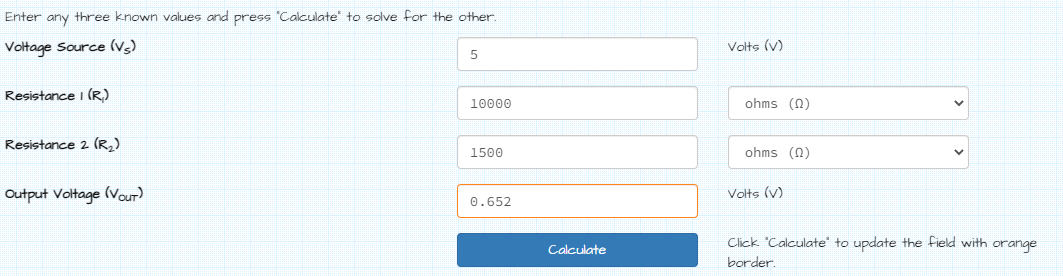Now we repeat with the 4555.556ohm in the resistance 2 box, clear out the output voltage box and hit calculate again.
It will give us 1.565v.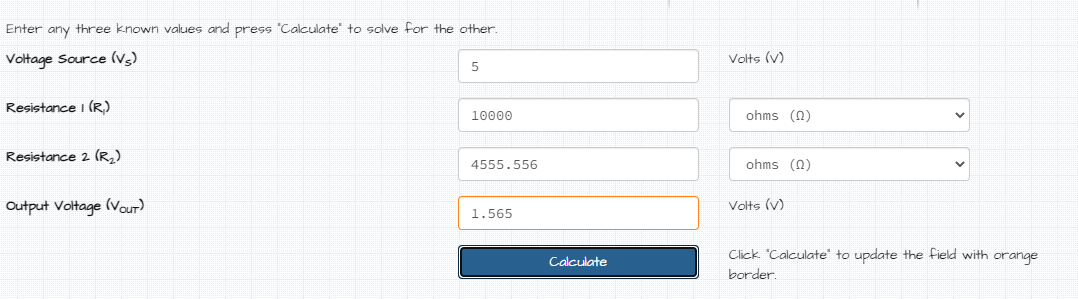So now we know that with a 10k pull up to 5v, the new calibration is the following:
.651v = -30c
1.565v = 150c

We can now input this calibration into the Haltech and use the SPI for our oil temperature sensor.
If you have any farther questions just send an email to Support@Haltech.com.

• # Related Articles

• ## Ambient Air Temperature Sensor

An Ambient Air Temperature Sensor measures the temperature of the air outside of the vehicle. Temperature sensors are typically two-wire sensors; a signal wire that is connected to an AVI, and a signal ground wire. On this node, the Ambient Air ...
• ## Fuel Temperature Sensor

A Fuel Temperature Sensor can be used with your ECU for datalogging and for Engine Protection purposes. Temperature sensors are typically two wire sensors; a signal wire that is connected to an AVI, and a signal ground wire. Fuel Temperature Sensor ...
• ## Air Conditioner Temperature Sensor

An Air Conditioner Temperature Sensor allows the ECU to continuously monitor the temperature of the air conditioning system. Temperature sensors are typically two wire sensors; a signal wire that is connected to an AVI, and a signal ground wire.+ Air ...
• ## Intake Air Temperature Sensor

An Intake Air Temperature Sensor measures the temperature of the incoming air to the engine. This information is provided to the ECU to determine the correct amount of fuel needed for optimum performance and economy. The information from this sensor ...
• ## Temperature Sensor Problem Diagnosis

What is a Temperature Sensor A temperature sensor is actually a resistor that changes its resistance depending on temperature, typically using something called a thermistor. It is this resistance change that can be used by the ECU to interpret ...